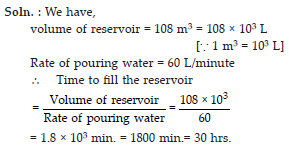Mensuration (Mathematics) Class 8 - NCERT Questions
Q 1.

A square and a rectangular field with measurements as given in the figure have the same perimeter. Which field has a larger area ?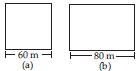SOLUTION:

We have, a square of side 60 m i.e., s = 60 m and a rectangle of length a = 80 m Perimeter of square = 4 × s = 4 × 60 m = 240 m As given, the perimeter of square and rectangle are equal.
Let, b be the other side of a rectangle.
2 × a + 2 × b = 240 m
⇒ 2 × 80 + 2 × b = 240
⇒ 2 × b = 240 – 160 ⇒ 2 × b = 80 m
⇒ b = 40 m
Hence, area of a square = s2 = 60 m × 60 m = 3600 sq. m
Area of rectangle = a × b = 80 m × 40 m = 3200 sq. m
Hence, area of a square is larger than that of the rectangle.

Q 2.

Mrs. Kaushik has a square plot with the measurement as shown in figure. She wants to construct a house in the middle of the plot. A garden is developed around the house. Find the total cost of developing a garden around the house at the rate of Rs. 55 per m2.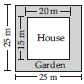SOLUTION:

The dimensions of the plot and house are as shown.
The area of plot = 25 × 25 = 625 sq. m
and the area of house = 20 × 15 = 300 sq. m
We know, Area of plot = Area of house + Area of garden
Area of garden = Area of plot – Area of house =625 – 300 = 325 sq. m
We also know,
Rate of developing 1 sq. m garden = Rs. 55
Amount for developing 325 sq. m garden =325 × 55 = Rs. 17875.

Q 3.

The shape of a garden is rectangular in the middle and semi circular at the ends as shown in the diagram. Find the area and the perimeter of this garden (Length of rectangle is 20 – (3.5 + 3.5) metres).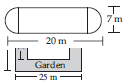SOLUTION: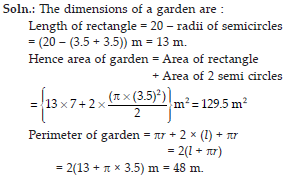Q 4.

A flooring tile has the shape of a parallelogram whose base is 24 cm and the corresponding height is 10 cm. How many such tiles are required to cover a floor of area 1080 m2? (If required you can split the tiles in whatever way you want to fill up the corners).

SOLUTION: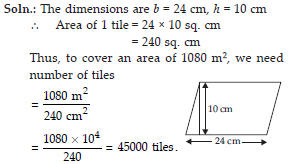Q 5.

An ant is moving around a few food pieces of different shapes scattered on the floor. For which food-piece would the ant have to take a longer round ? Remember, circumference of a circle can be obtained by using the expression c = 2pr, where r is the radius of the circle.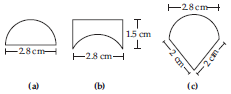SOLUTION: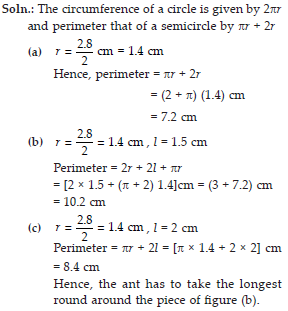Q 6.

The shape of the top surface of a table is a trapezium. Find its area if its parallel sides are 1 m and 1.2 m and perpendicular distance between them is 0.8 m.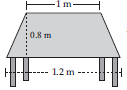SOLUTION:

Area of trapezium =×12 (Sum of the parallel sides) × Distance between parallel sides.
=1/2×(1+2.1)×(0.8)m2
=1/2 (2.2)×(0.8)m2=0.88m2

Q 7.

The area of a trapezium is 34 cm2 and the length of one of the parallel sides is 10 cm and its height is 4 cm. Find the length of the other parallel side.

SOLUTION: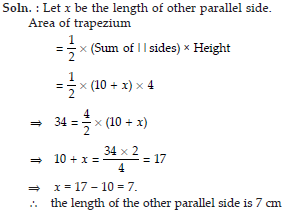Q 8.

Length of the fence of a trapezium shaped field ABCD is 120 m. If BC = 48 m, CD = 17m and AD = 40 m, find the area of this field. Side AB is perpendicular to the parallel sides AD and BC.

SOLUTION: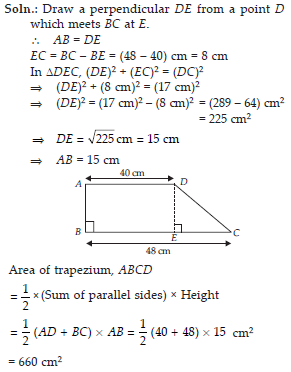Q 9.

The diagonal of a quadrilateral shaped field is 24 m and the perpendiculars dropped on it from the remaining opposite vertices are 8 m and 13 m. Find the area of the field.

SOLUTION: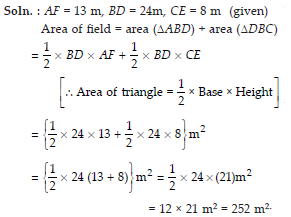Q 10.

The diagonals of a rhombus are 7.5 cm and 12 cm. Find its area.

SOLUTION: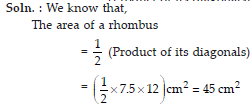Q 11.

Find the area of a rhombus whose side is 6 cm and whose altitude is 4 cm. If one of its diagonals is 8 cm long, find the length of the other diagonal.

SOLUTION:

Since, rhombus is a parallelogram whose all sides are equal.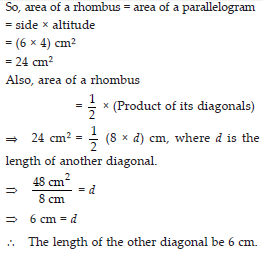Q 12.

The floor of a building consists of 3000 tiles which are rhombus shaped and each of its diagonals are 45 cm and 30 cm in length. Find the total cost of polishing the floor, if the cost per m2 is Rs.4.

SOLUTION: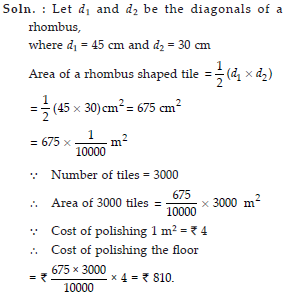Q 13.

Mohan wants to buy a trapezium shaped field. Its side along the river is parallel to and twice the side along the road. If the area of this field is 10500 m2 and the perpendicular distance between the two parallel sides is 100 m, find the length of the side along the river.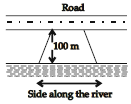SOLUTION: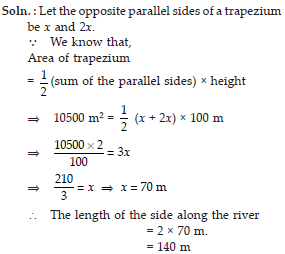Q 14.

Top surface of a raised platform is in the shape of a regular octagon as shown in the figure. Find the area of the octagonal surface.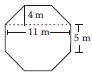SOLUTION: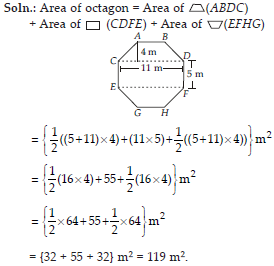Q 15.

There is a pentagonal shaped park as shown in the figure. For finding its area Jyoti and Kavita divided it in two different ways. Find the area of this park using both ways. Can you suggest some other way of finding its area ?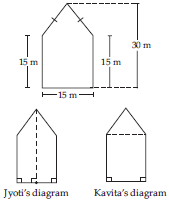SOLUTION: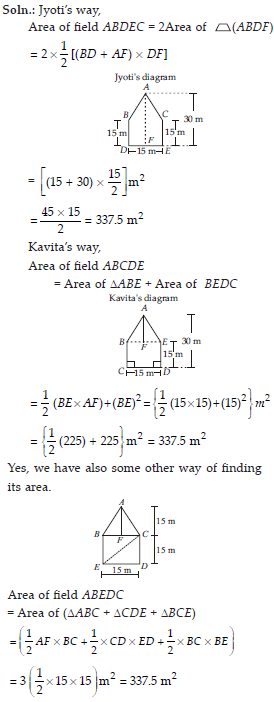Q 16.

Diagram of the adjacent picture frame has outer dimensions = 24 cm × 28 cm and inner dimensions = 16 cm × 20 cm. Find the area of each section of the frame, if the width of each section is same.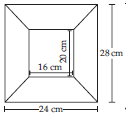SOLUTION: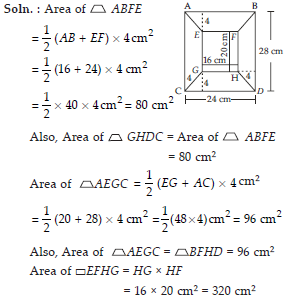Q 17.

There are two cuboidal boxes as shown in the adjoining figure. Which box requires the lesser amount of material to make ?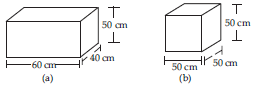SOLUTION:

Surface area of box (a) = 2(lb + bh + hl)
= 2(60 × 40 + 40 × 50 + 50 × 60)
= 2(2400 + 2000 + 3000)
= 2 × 7400
= 14800 cm2
Surface area of box (b) = 6 × (side)2
= 6 × 502
= 15000 cm2
Hence, box (a) required less amount of material than box (b) to make.

Q 18.

A suitcase with measures 80 cm × 48 cm × 24 cm is to be covered with a trapaulin cloth. How many metres of trapaulin of width 96 cm is required to cover 100 such suitcases ?

SOLUTION: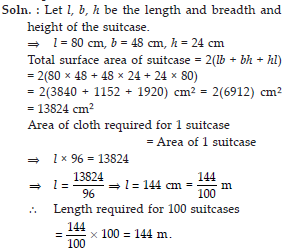Q 19.

Find the side of a cube whose surface area is 600 cm2.

SOLUTION: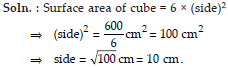Q 20.

Rukhsar painted the outside of the cabinet of measure 1 m × 2 m × 1.5 m. How much surface area did she cover if she painted all except the bottom of the cabinet.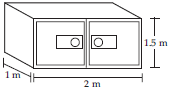SOLUTION:

Rukhsar painted all the cabinet except the bottom means she painted 4 walls and 1 top. Let l = 1 m, b = 2 m, h = 1.5 m be the length, breadth and height of cabinet.
Area of painted cabinet
= (lb + bh + bh + lh + lh)
= [1 × 2 + 2 × 1.5 + 2 × 1.5 + 1 × 1.5 + 1 × 1.5] m2
= [2 + 3.0 + 3.0 + 1.5 + 1.5] m2 = 11 m2.

Q 21.

Daniel is painting the walls and ceiling of a cuboidal hall with length, breadth and height of 15 m, 10 m and 7 m respectively. From each can of paint 100 m2 of area is painted. How many cans of paints will she need to paint the room ?

SOLUTION:

Soln. : For painting the walls and ceiling means Daniel is painting 4 walls and 1 ceiling.
Total painted area = Area of 4 walls + Area of ceiling
Let l = 15, b = 10 and h = 7 be the length, breadth and height of hall.
= 2 × h(l + b) + lb = {2 × 7 (15 + 10) + (15 × 10)} m2
= {14 (25) + 150} m2 = {350 + 150} m2 = 500 m2 If 100 m2 of area is painted with one can
500 m2 of area is painted with 500/100 cans = 5 cans.

Q 22.

Describe how the two figures at the right are alike and how they are different. Which box has larger lateral surface area?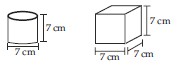SOLUTION:

Alike : They have the same dimensions. Different : Their shapes are different. Let r and h be the radius and height of the cylinder,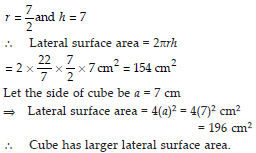Q 23.

A closed cylindrical tank of radius 7 m and height 3 m is made from a sheet of metal. How much sheet of metal is required ?

SOLUTION: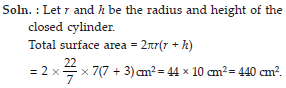Q 24.

The lateral surface area of a hollow cylinder is 4224 cm2. It is cut along its height and formed a rectangular sheet of width 33 cm. Find the perimeter of rectangular sheet ?

SOLUTION: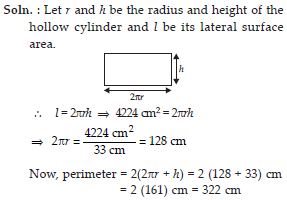Q 25.

A road roller takes 750 complete revolutions to move once over to level a road. Find the area of the road if the diameter of a raod roller is 84 cm and length is 1 m.

SOLUTION: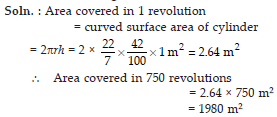Q 26.

A company packages its milk powder in cylindrical container whose base has a diameter of 14 cm and height 20 cm. Company places a label around the surface of the container (as shown in figure). If the label is placed 2 cm from top and bottom, what is the area of the label.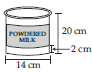SOLUTION: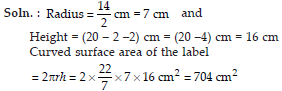Q 27.

Given a cylindrical tank, in which situation will you find surface area and in which situation volume.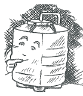(a) To find how much it can hold.
(b) Number of cement bags required to plaster it.
(c) To find the number of smaller tanks that can be filled with water from it.

SOLUTION:

(a) To find how much a cylinder can hold, we need to find the volume of the cylindrical tank.
(b) To find the number of cement bags required to plaster the tank, we need to find the surface area of the cylindrical tank.
(c) To find the number of smaller tanks that can be filled with water from the bigger tank, we need to find the volume of the big cylindrical tank and one small tank.

Q 28.

Diameter of cylinder A is 7 cm, and the height is 14 cm. Diameter of cylinder B is 14 cm and height is 7 cm. Without doing any calculations can you suggest whose volume is greater ? Verify it by finding the volume of both the cylinder. Check whether the cylinder with greater volume also has greater surface area?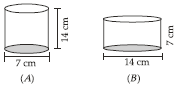SOLUTION: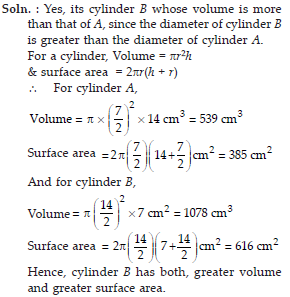Q 29.

Find the height of a cuboid whose base area is 180 cm2 and volume is 900 cm3?

SOLUTION: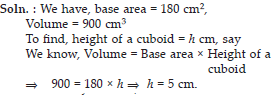Q 30.

A cuboid is of dimensions 60 cm × 54 cm × 30 cm. How many small cubes with side 6 cm can be placed in the given cuboid ?

SOLUTION: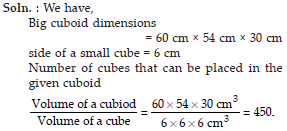Q 31.

Find the height of the cylinder whose volume is 1.54 m3 and diameter of the base is 140 cm ?

SOLUTION: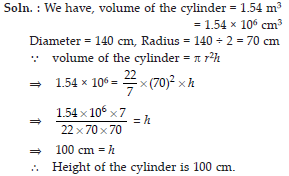Q 32.

A milk tank is in the form of cylinder whose radius is 1.5 m and length is 7 m. Find the quantity of milk in litres that can be stored in the tank ?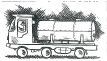SOLUTION: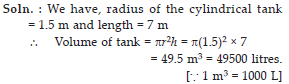Q 33.

If each edge of a cube is doubled,
(i) how many times will its surface area increase?
(ii) how many times will its volume increase?

SOLUTION: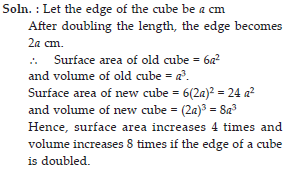Q 34.

Water is pouring into a cuboidal reservoir at the rate of 60 litres per minute. If the volume of reservoir is 108 m3, find the number of hours it will take to fill the reservoir.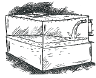SOLUTION: